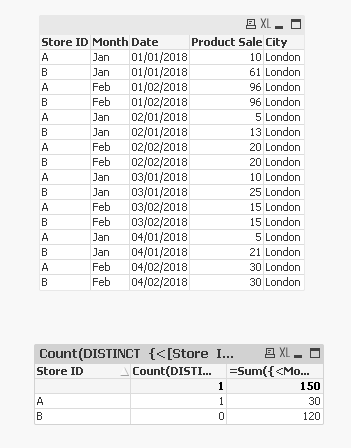# New to QlikView

Discussion board where members can get started with QlikView.MVP

It does sum up at the store level, but assuming that there is only one city and one month to look at

New Contributor III

Hi Sunny - I have just tried the logic and obtain an error in expression. I think it is not recognising the SUM function because of the "= before it. ie The font colour of the sum function is not blue. Is there a way to fix this? Thanks.MVP

Count(DISTINCT {<[Store ID] = {"=Sum({<Month = {'Jan'}, City = {'London'}>} [Product Sale]) < 50"}, Month = {'Jan'}, City = {'London'}>}[Store ID])New Contributor III

Works perfectly! Thanks Sunny!

HighlightedMVP

SuperNew Contributor III

Hi Sunny,

Sorry to bother you again. I have forgotten to add a second condition in that logic. I need to count the number of distinct Store ID if:

(1) the store has sold less than 50 items (Product Sale < 50 ) - Already covered

(2) and has been open for more than 3 days.

I was thinking of adding another condition in the one you posted. Something like max(Date) - min(Date) > 3 for each Store ID. Is it possible to embed such a logic within the one you showed me. If so, where would it be placed?

Many thanks,

RKMVP

May be this

Count(DISTINCT {<[Store ID] = {"=Sum({<Month = {'Jan'}, City = {'London'}>} [Product Sale]) < 50 and max(Date) - min(Date) > 3"}, Month = {'Jan'}, City = {'London'}>}[Store ID])

New Contributor III

Will it take account the other months? In this case the min date would be 01/01/2018 and max date would be 04/02/2018. Because you set the month to be Jan in the set analysis, will it take the other months into consideration? Many thanksMVP